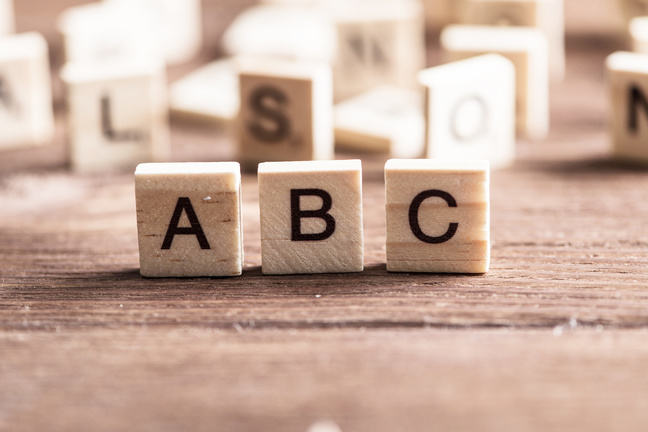## Want to keep learning?

This content is taken from the University of Padova's online course, Precalculus: the Mathematics of Numbers, Functions and Equations. Join the course to learn more.
1.4

## PrecalculusGlossary

# Glossary

We are planning to prepare a Glossary that contains a list of the key terms that are used in the course. Many of them are defined in the next steps. Please refer to this section, when you find some unknown term.

Which terms would you like us to explain here?

## Symbols

$$:=$$ Equal by definition. E.g., for any real number $$a\in \mathbb R$$ one has $$a^2:= a\cdot a$$.

$$\exists$$ :There exists.

$$\in$$: Belongs to

$$\subset$$: Is a subset of

$$\forall$$: For every

$$\mathbb N$$: The set of natural numbers $$0,1,2,3,…$$.

$$\mathbb Q$$: The set of rational numbers.

$$\mathbb R$$: The set of real numbers.

$$\mathbb Z$$: The set of integer numbers $$…,-3,-2,-1,0, 1,2,3,…$$: $$\mathbb N\subset\mathbb Z\subset\mathbb Q\subset\mathbb R$$

$$\cup$$: Union of sets. For instance if $$A=\lbrace1,2,3\rbrace, \, B=\lbrace3,4,5\rbrace$$ then $$A\cup B=\lbrace1,2,3,4,5\rbrace$$.

$$\cap$$: Intersection of sets. For instance if $$A=\lbrace 1,2,3\rbrace, \, B=\lbrace3,4,5\rbrace$$ then $$A\cap B=\lbrace3\rbrace$$.

## I

Integer: a number of the set $$\mathbb Z=\lbrace …,-3,-2,-1,0,1,2,3,…\rbrace$$

## N

Natural number: a number of the set $$\mathbb N=\lbrace 0,1,2,3,…\rbrace$$

## R

Rational number: a number of the set $$\mathbb Q=\left\lbrace \dfrac ab:\, a\in\mathbb Z, b\in\mathbb Z\setminus\lbrace 0\rbrace\right\rbrace=\left\lbrace \dfrac ab:\, a\in\mathbb Z, b\in\mathbb N\setminus\lbrace 0\rbrace\right\rbrace$$

Real number: an element of $$\mathbb R$$. The set $$\mathbb R$$ contains the limits of sequences of rational numbers and elements like square roots, $$\pi, e,…$$

## S

Square root of $$x\ge 0$$: the positive number $$y$$ satisfying $$y^2=x$$.

$$m-$$th root of $$x\ge 0$$: the positive number $$y$$ satisfying $$y^m=x$$.